Courses

Test: 35 Year JEE Previous Year Questions: Mechanical Properties of Solids and Fluids

30 Questions MCQ Test Physics 35 Years JEE Main & Advanced Past year Papers | Test: 35 Year JEE Previous Year Questions: Mechanical Properties of Solids and Fluids

Description
Attempt Test: 35 Year JEE Previous Year Questions: Mechanical Properties of Solids and Fluids | 30 questions in 60 minutes | Mock test for JEE preparation | Free important questions MCQ to study Physics 35 Years JEE Main & Advanced Past year Papers for JEE Exam | Download free PDF with solutions
QUESTION: 1

A cylindrical tank has a hole of diameter 2r in its bottom. The hole is covered wooden cylindrical block of diameter 4r, height h and density ρ/3. Situation I : Initially, the tank is filled with water of density ρ to a height such that the height of water above the top of the block is h1 (measured from the top of the block). Situation II : The water is removed from the tank to a height h2 (measured from the bottom of the block), as shown in the figure. The height h2 is smaller than h (height of the block) and thus the block is exposed to the atmosphere.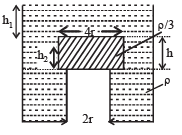Q. Find the minimum value of height h1 (in situation 1), for which the block just starts to move up?

Solution:

Consider the equilibrium of wooden block.
Forces acting in the downward direction are

(a) Weight of wooden cylinder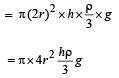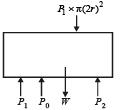(b) Force due to pressure (P1) created by liquid of height h1 above the wooden block is
= P1 × π (2r)2 = [P0 + h1rg] × π (2r)2
Force acting on the upward direction due to pressure P2 exerted from below the wooden block and atmospheric pressure is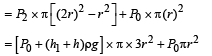At the verge of rising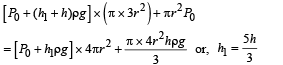QUESTION: 2

A cylindrical tank has a hole of diameter 2r in its bottom. The hole is covered wooden cylindrical block of diameter 4r, height h and density ρ/3. Situation I : Initially, the tank is filled with water of density ρ to a height such that the height of water above the top of the block is h1 (measured from the top of the block). Situation II : The water is removed from the tank to a height h2 (measured from the bottom of the block), as shown in the figure. The height h2 is smaller than h (height of the block) and thus the block is exposed to the atmosphere.Q. Find the height of the water level h2 (in situation 2), for which the block remains in its original position without the application of any external force

Solution:

KEY CONCEPT : Considering equilibrium of wooden block.
Total downward force = Total force upwards Wt. of block + force due to atmospheric pressure = Force due to pressure of liquid + Force due to atmospheric pressure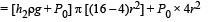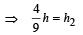QUESTION: 3

A cylindrical tank has a hole of diameter 2r in its bottom. The hole is covered wooden cylindrical block of diameter 4r, height h and density ρ/3. Situation I : Initially, the tank is filled with water of density ρ to a height such that the height of water above the top of the block is h1 (measured from the top of the block). Situation II : The water is removed from the tank to a height h2 (measured from the bottom of the block), as shown in the figure. The height h2 is smaller than h (height of the block) and thus the block is exposed to the atmosphere.Q. In situation 2, if h2 is further decreased, then

Solution:

When the height h2 of water level is further decreased, then the upward force acting on the wooden block decreases. The total force downward remains the same.
This difference will be compensated by the normal reaction by the tank wall on the wooden block. Thus the block does not moves up and remains at its original position.

QUESTION: 4

When liquid medicine of density ρ is to put in the eye, it is done with the help of a dropper. As the bulb on the top of the dropper is pressed, a drop forms at the opening of the dropper. We wish to estimate the size of the drop. We first assume that the drop formed at the opening is spherical because that requires a minimum increase in its surface energy. To determine the size, we calculate the net vertical force due to the surface tension T when the radius of the drop is R. When this force becomes smaller than the weight of the drop, the drop gets detached from the dropper.

Q. If the radius of the opening of the dropper is r, the vertical force due to the surface tension on the drop of radius R (assuming r << R) is

Solution:

The vertical force due to surface tension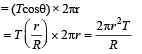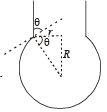QUESTION: 5

When liquid medicine of density ρ is to put in the eye, it is done with the help of a dropper. As the bulb on the top of the dropper is pressed, a drop forms at the opening of the dropper. We wish to estimate the size of the drop. We first assume that the drop formed at the opening is spherical because that requires a minimum increase in its surface energy. To determine the size, we calculate the net vertical force due to the surface tension T when the radius of the drop is R. When this force becomes smaller than the weight of the drop, the drop gets detached from the dropper.

Q. If r = 5 × 10–4 m, ρ = 103 kgm–3 , g = 10 ms–2 ,T= 0.11Nm –1, the radius of the drop when it detaches from the dropper is approximately

Solution:

When the drop is about to detach from the dropper Weight = vertical force due to surface tension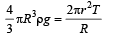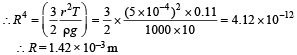QUESTION: 6

When liquid medicine of density ρ is to put in the eye, it is done with the help of a dropper. As the bulb on the top of the dropper is pressed, a drop forms at the opening of the dropper. We wish to estimate the size of the drop. We first assume that the drop formed at the opening is spherical because that requires a minimum increase in its surface energy. To determine the size, we calculate the net vertical force due to the surface tension T when the radius of the drop is R. When this force becomes smaller than the weight of the drop, the drop gets detached from the dropper.

Q. After the drop detaches, its surface energy is

Solution: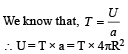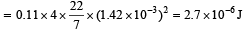QUESTION: 7

A spray gun is shown in the figure where a piston pushes air out of a nozzle. A thin tube of uniform cross section is connected to the nozzle. The other end of the tube is in a small liquid container.
As the piston pushes air through the nozzle, the liquid from the container rises into the nozzle and is sprayed out. For the spray gun shown, the radii of the piston and the nozzle are 20 mm and 1 mm respectively. The upper end of the container is open to the atmosphere.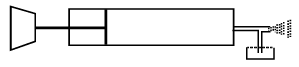Q. If the piston is pushed at a speed of 5 mms–1, the air comes out of the nozzle with a speed of

Solution:

From principle of continuity,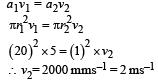QUESTION: 8

A spray gun is shown in the figure where a piston pushes air out of a nozzle. A thin tube of uniform cross section is connected to the nozzle. The other end of the tube is in a small liquid container.
As the piston pushes air through the nozzle, the liquid from the container rises into the nozzle and is sprayed out. For the spray gun shown, the radii of the piston and the nozzle are 20 mm and 1 mm respectively. The upper end of the container is open to the atmosphere.

Q. If the density of air is ρa, and that of the liquid ρl, then for a given piston speed the rate (volume per unit time) at which the liquid is sprayed will be proportional to

Solution: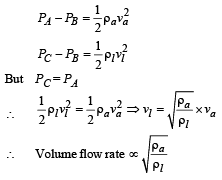QUESTION: 9

STATEMENT-1 : The stream of water flowing at high speed from a garden hose pipe tends to spread like a fountain when held vertically up, but tends to narrow down when held vertically down.
STATEMENT-2 : In any steady flow of an incompressible fluid, the volume flow rate of the fluid remains constant.

Solution:

We know that volume flow rate (V) of an incompressible fluid in steady flow remains constant.

V = a × v

where a = area of cross-section and  v =  velocity

⇒ If v decreases a increases and vice - versa.
When stream of water moves up, its speed (v) decreases and therefore 'a' increases i.e. the water spreads out as a fountain. When stream of water from hose pipe moves down, its speed increases and therefore area of crosssection decreases.
Therefore statement-1 is true and statement-2 is the correct explanation of statement-1.

QUESTION: 10

A spring of force constant 800 N/m has an extension of 5 cm. The work done in extending it from 5 cm to 15 cm is

Solution:

Small amount of work done in extending the spring by dx is

dW = k x dx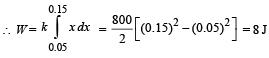QUESTION: 11

A wire fixed at the upper end stretches by length ℓ by applying a force F. The work done in stretching is

Solution:

Work done by constant force in displacing the object by a distance ℓ.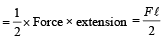QUESTION: 12

Spherical balls of radius ‘R’ are falling in a viscous fluid of viscosity ‘η’ with a velocity ‘v’. The retarding viscous force acting on the spherical ball is

Solution:

From Stoke's law,
viscous force F = 6πηrv
hence F is directly proportional to radius & velocity.

QUESTION: 13

If two soap bubbles of  different radii are connected by a tube.

Solution: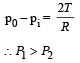hence air moves from smaller bubble to bigger bubble.

QUESTION: 14

If ‘S’ is stress and ‘Y’ is young’s modulus of material of a wire, the energy stored in the wire per unit volume is

Solution:

Energy stored per unit volume,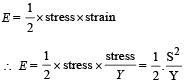QUESTION: 15

A 20 cm long capillary tube is dipped in water. The water rises up to 8 cm. If the entire arrangement is put in a freely falling elevator the length of water column in the capillary tube will be

Solution:

Water fills the tube entirely in gravity less condition i.e., 20 cm.

QUESTION: 16

A wire elongates by l mm when a load W is hanged from it.
If the wire goes over a pulley and two weights W each are hung at the two ends, the elongation of the wire will be (in mm)

Solution:

Case (i)

At equilibrium, T = W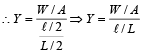Case (ii)   At equilibrium T = W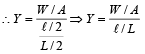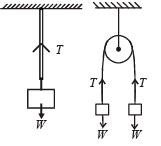⇒ Elongation is the same.

QUESTION: 17

If the terminal speed of a sphere of gold (density = 19.5 kg/m3) is 0.2 m/s in a viscous liquid (density = 1.5 kg/m3), find the terminal speed of a sphere of silver (density = 10.5 kg/m3) of the same size in the same liquid

Solution: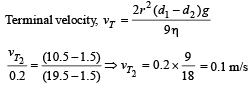QUESTION: 18

A spherical solid ball of volume V is made of a material of density ρ1. It is falling through a liquid of density ρ22< ρ1). Assume that the liquid applies a viscous force on the ball that is proportional to the square of its speed v, i.e., Fviscous = –kv2 (k > 0). The terminal speed of the ball is

Solution:

The condition for terminal speed (vt) is Weight = Buoyant force + Viscous force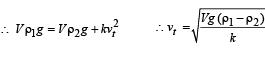QUESTION: 19

A jar is filled with two non-mixing liquids 1 and 2 having densities ρ1 and, ρ2 respectively. A solid ball, made of a material of density ρ3, is dropped in the jar. It comes to equilibrium in  the position shown in the figure.Which of the following is true for ρ1, ρand ρ3?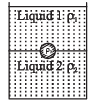Solution:

From the figure it is clear that liquid 1 floats on liquid  2.
The lighter liquid floats over heavier liquid. Therefore we can conclude that ρ1 < ρ2

Also ρ3 < ρ2 otherwise the ball would have sink to the bottom of the jar.
Also ρ3 > ρ1 otherwise the ball would have floated in liquid 1. From the above discussion we conclude that ρ1 < ρ3 < ρ2.

QUESTION: 20

A capillary tube (A) is dipped in water. Another identical tube (B) is dipped in a soap-water solution. Which of the following shows the relative nature of the liquid columns in the two tubes?

Solution:

In case of water, the meniscus sh ape is concave upwards. Also according to ascent formula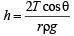The surface tension (T) of  soap solution is less than water. Therefore rise of soap solution in the capillary tube is less as compared to water. As in the case of water, the meniscus shape of soap solution is also concave upwards.

QUESTION: 21

Two wires are made of the same material and have the same volume. However wire 1 has cross-sectional area A and wire 2 has cross-sectional area 3A. If the length of wire 1 increases by Δx on applying force F, how much force is needed to stretch wire 2 by the same amount?

Solution: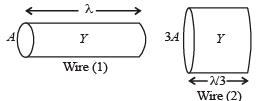As shown in the figure, the wires will have the same Young’s modulus (same material) and the length of the wire of area of cross-section 3A will be  ℓ/3 (same volume as wire 1).
For wire 1,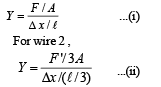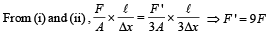QUESTION: 22

A ball is made of a material of density ρ where ρoil <ρ< ρwater with ρoil and ρwater representing the densities of oil and water, respectively. The oil and water are immiscible. If the above ball is in equilibrium in a mixture of this oil and water, which of the following pictures represents its equilibrium position?

Solution:

Oil will float on water so, (2) or (4) is the correct option.
But density of ball is more than that of oil,, hence it will sink in oil.

QUESTION: 23

Two identical charged spheres are suspended by strings of equal lengths. The strings make an angle of 30° with each other. When suspended in a liquid of density 0.8g cm–3, the angle remains the same. If density of the material of the sphere is 1.6 g cm–3 , the dielectric constant of the liquid is

Solution: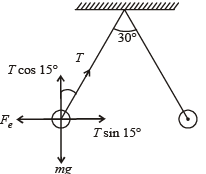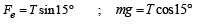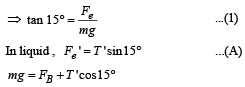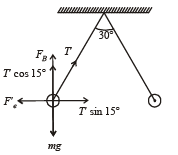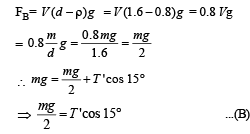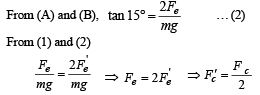QUESTION: 24

Work done in increasing the size of a soap bubble from a radius of 3 cm to 5 cm is nearly (Surface tension of soap solution = 0.03 Nm–1)

Solution:

W = T × change in surface area
W = 2T4π[(52) – (3)2] × 10–4
= 2 × 0.03 × 4π [25 – 9] × 10–4 J = 0.4π × 10–3 J
= 0.4π mJ

QUESTION: 25

Water is flowing continuously from a tap having an internal diameter 8 × 10–3 m. The water velocity as it leaves the tap is 0.4 ms–1. The diameter of the water stream at a distance 2 × 10–1 m below the tap is close to:

Solution:

From Bernoulli's theorem,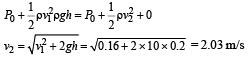From equation of continuity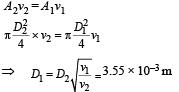QUESTION: 26

A thin liquid film formed between a U-shaped wire and a light slider supports a weight of 1.5 × 10–2 N  (see figure). The length of the slider is 30 cm and its weight negligible. The surface tension of the liquid film is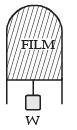Solution:

At equilibrium,

2Tl = mg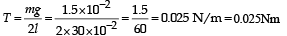QUESTION: 27

A unifor m cylinder of length L and mass M having crosssectional area A is suspended, with its length vertical, from a fixed point by a massless spring such that it is half submerged in σ liquid of density s at equilibrium position. The extension x0 of the spring when it is in equilibrium is:

Solution:

From figure,  kx0 + FB = Mg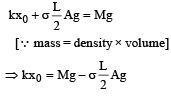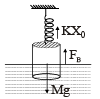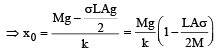QUESTION: 28

Assume that a drop of liquid evaporates by decrease in its surface energy, so that its temperature remains unchanged.What should be the minimum radius of the drop for this to be possible? The surface tension is T, density of liquid is ρ and L is its latent heat of vaporization.

Solution: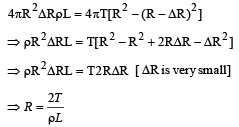QUESTION: 29

On heating water, bubbles being formed at the bottom of the vessel detach and rise. Take the bubbles to be spheres of radius R and making a circular contact of radius r with the bottom of the vessel. If r << R and the surface tension of water is T, value of r just before bubbles detach is: (density of water is ρ w)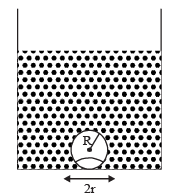Solution:

(None) None of the given option is correct.
When the bubble gets detached, Buoyant force = force due to surface tension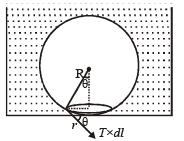Force due to excess pressure =  upthrust Access pressure in air bubble = 2T/R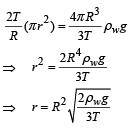QUESTION: 30

An open glass tube is immersed in mercury in such a way that a length of 8 cm extends above the mercury level. The open end of the tube is then closed and sealed and the tube is raised vertically up by additional 46 cm. What will be length of the air column above mercury in the tube now? (Atmospheric pressure = 76 cm of Hg)

Solution: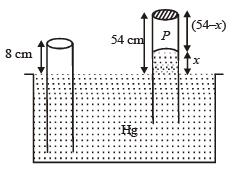Length of the air column above mercury in the tube is, P + x = P0

⇒ P = (76 – x)
⇒ 8 × A × 76 = (76 – x) × A × (54 – x)
∴ x = 38

Thus, length of air column = 54 – 38 = 16 cm.Use Code STAYHOME200 and get INR 200 additional OFF Use Coupon Code

Track your progress, build streaks, highlight & save important lessons and more!

Similar ContentRelated tests